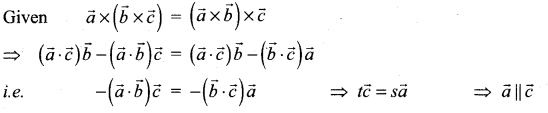## Tamilnadu Samacheer Kalvi 12th Maths Solutions Chapter 6 Applications of Vector Algebra Ex 6.10

Choose the correct or the most suitable answer from the given four alternatives:
Question 1.
If $$\vec{a}$$ and $$\vec{b}$$ are parallel vector, then $$[\vec{a}, \vec{c}, \vec{b}]$$ is equal to ………………..
(a) 2
(b) -1
(c) 1
(d) 0
Solution:
(d) 0
Hint:
$$\vec{a}$$ and $$\vec{b}$$ are parallel vectors, so $$\vec{a} \times \vec{b}$$ = 0
then $$[\vec{a} \vec{c} \vec{b}]=-[\vec{a} \vec{b} \vec{c}]=-(\vec{a} \times \vec{b}) \cdot \vec{c}$$ = 0

Question 2.
If a vector $$\vec{\alpha}$$ lies in the plane of $$\vec{\beta}$$ and $$\vec{\gamma}$$, then …………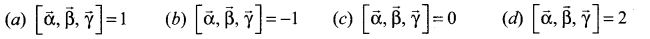Solution:
(c) $$[\vec{\alpha}, \vec{\beta}, \vec{\gamma}]$$ = 0
Hint:
Since $$[\vec{\alpha}, \vec{\beta}, \vec{\gamma}]$$ are lie in the same plane
so $$[\vec{\alpha}, \vec{\beta}, \vec{\gamma}]$$ = 0

Question 3.
If $$\vec{a} \cdot \vec{b}=\vec{b} \cdot \vec{c}=\vec{c} \cdot \vec{a}$$= 0, then the value of $$|[\vec{a}, \vec{b}, \vec{c}]|$$ is ……………….
(a) $$|\vec{a}||\vec{b}||\vec{c}|$$
(b) $$\frac{1}{3}|\vec{a}||\vec{b}||\vec{c}|$$
(c) 1
(d) -1
Solution:
(a) $$|\vec{a}||\vec{b}||\vec{c}|$$
Hint: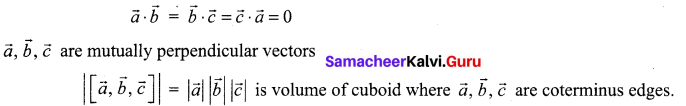Question 4.
If $$\vec{a}, \vec{b}, \vec{c}$$ are three unit vectors such that $$\vec{a}$$ is perpendicular to $$\vec{b}$$, and is parallel to $$\vec{c}$$ then $$\vec{a} \times(\vec{b} \times \vec{c})$$is equal to ………………….
(a) $$\vec{a}$$
(b) $$\vec{b}$$
(c) $$\vec{c}$$
(d) $$\vec{0}$$
Solution:
(b) $$\vec{b}$$
Hint: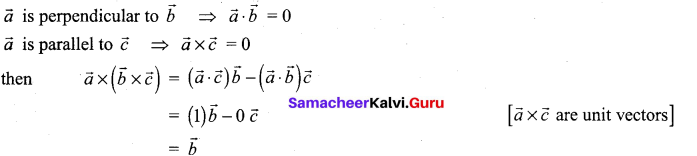Question 5.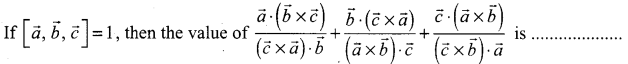(a) 1
(b) -1
(c) 2
(d) 3
Solution:
(a) 1
Hint: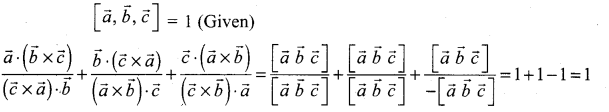Question 6.
The volume of the parallelepiped with its edges represented by the vectors $$\hat{i}+\hat{j}, i+2 \hat{j}$$, $$\hat{i}+\hat{j}+\pi \hat{k}$$ is ……………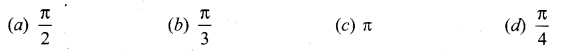Solution:
(c) π
Hint:
Volume = $$[\vec{a} \vec{b} \vec{c}]=\left|\begin{array}{ccc}{1} & {1} & {0} \\ {1} & {2} & {0} \\ {1} & {1} & {\pi}\end{array}\right|$$
= π(2 – 1) = π cubic unitsQuestion 7.
If $$\vec{a}$$ and $$\vec{b}$$ are unit vectors such that $$[\vec{a}, \vec{b}, \vec{a} \times \vec{b}]=\frac{\pi}{4}$$ then the angle between $$\vec{a}$$ and $$\vec{b}$$ is …………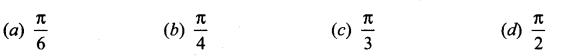Solution:
(a) $$\frac{\pi}{6}$$
Hint: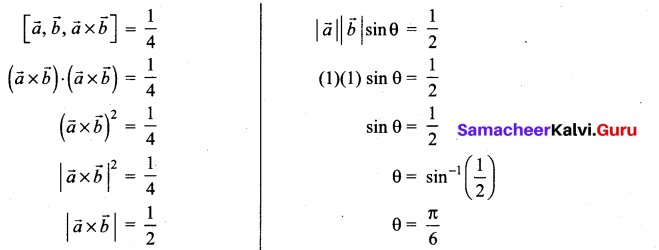Question 8.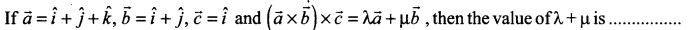(a) 0
(b) 1
(c) 6
(d) 3
Solution:
(a) 0
Hint: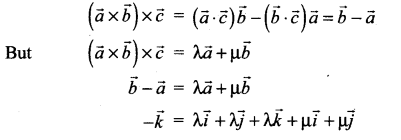Equate corresponding coefficients on both sides
λ + µ = 0 and λ = -1 this gives µ = 1
∴ Then the value of λ + µ = 0.

Question 9.
If $$\vec{a}, \vec{b}, \vec{c}$$ are three non-coplanar vectors such that $$\vec{a} \times(\vec{b} \times \vec{c})=\frac{\vec{b}+\vec{c}}{\sqrt{2}}$$, then the angle between $$\vec{a}$$ and $$\vec{b}$$ is …………
(a) 81
(b) 9
(c) 27
(d) 18
Solution:
(a) 81
Hint: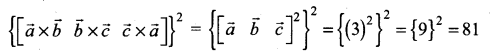Question 10.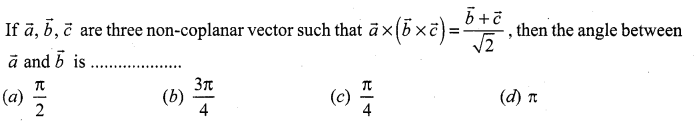Solution:
(b) $$\frac{3 \pi}{4}$$
Hint: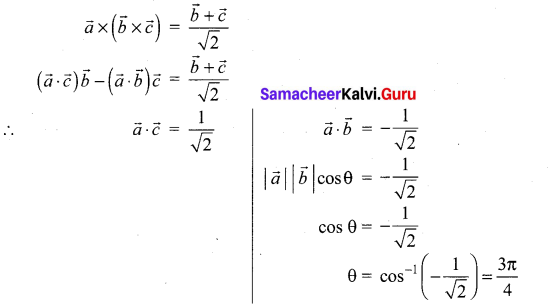Question 11.
If the volume of the parallelepiped with $$\vec{a} \times \vec{b}, \vec{b} \times \vec{c}, \vec{c} \times \vec{a}$$ as coterminous edges is 8 cubic units, then the volume of the parallelepiped with $$(\vec{a} \times \vec{b}) \times(\vec{b} \times \vec{c}),(\vec{b} \times \vec{c}) \times(\vec{c} \times \vec{a})$$ and $$(\vec{c} \times \vec{a}) \times(\vec{a} \times \vec{b})$$ as coterminous edges is ………………
(a) 8 cubic units
(b) 512 cubic units
(c) 64 cubic units
(d) 24 cubic units
Solution:
(c) 64 cubic units
Hint: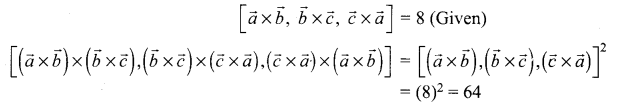Question 12.
Consider the vectors $$\vec{a}, \vec{b}, \vec{c}, \vec{d}$$ such that $$(\vec{a} \times \vec{b}) \times(\vec{c} \times \vec{d})=\overrightarrow{0}$$. Let P1 and P2 be the planes determined by the pairs of vectors $$\vec{a}, \vec{b}$$ and $$\vec{c}, \vec{d}$$ respectively. Then the angle between P1 and P2 is ……………..
(a) 0°
(b) 45°
(c) 60°
(d) 90°
Solution:
(a) 0°
Hint: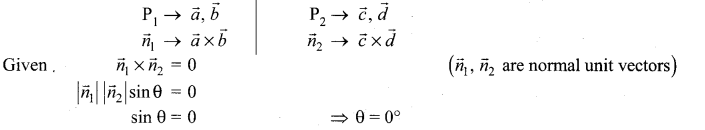Question 13.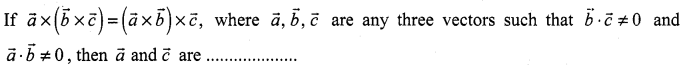(a) perpendicular
(b) parallel
(c) inclined at an angle $$\frac{\pi}{3}$$
(d) inclined at an angle $$\frac{\pi}{6}$$
Solution:
(b) parallel
Hint: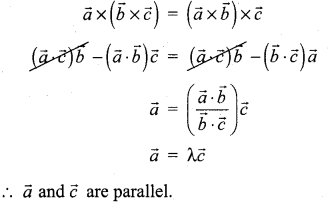Question 14.
If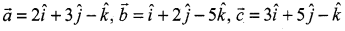, then a vector perpendicular to $$\vec{a}$$ and lies in the plane containing $$\vec{b}$$ and $$\vec{c}$$ is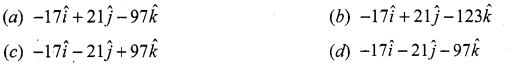Solution:
(d) $$-17 \hat{i}-21 \hat{j}-97 \hat{k}$$
Hint: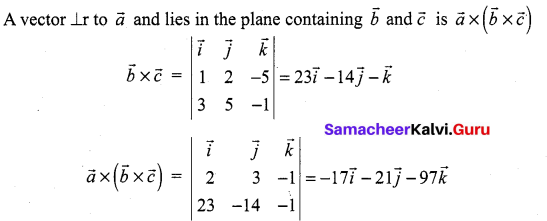Question 15.
The angle between the lines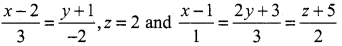is ………..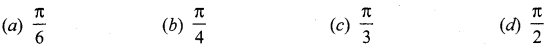Solution:
(d) $$\frac{\pi}{2}$$
Hint: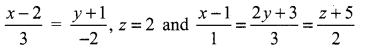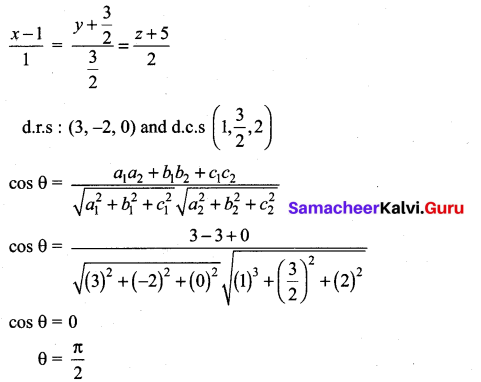Question 16.
If the line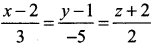lies in the plane x + 3 + αz + β = 0, then (α, β) is …………..
(a) (-5, 5)
(b) (-6, 7)
(c) (5, -5)
(d) (6, -7)
Solution:
(d) (-6, 7)
Hint:
d.c.s of the first line = (3, -5, 2)
d.c.s of the line perpendicular to plane = (1, 3, -α)
a1a2 + b1b2 + c1c2 = 0
3 – 15 – 2α = 0 => -12 – 2α = 0
-2α =12 => α = -6
Plane passes through the point (2, 1, -2) so
2 + 3 + 6(-2) + β = 0 => β = 7
(α, β) = (-6, 7)

Question 17.
The angle between the line $$\vec{r}=(\hat{i}+2 \hat{j}-3 \hat{k})+t(2 \hat{i}+\hat{j}-2 \hat{k})$$ and the plane $$\vec{r} \cdot(\hat{i}+\hat{j})+4$$ = 0 is ……………
(a) 0°
(b) 30°
(c) 45°
(d) 90°
Solution:
(c) 45°
Hint: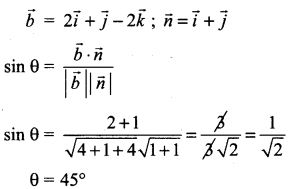Question 18.
The coordinates of the point where the line $$\vec{r}=(6 \hat{i}-\hat{j}-3 \hat{k})+t(-\hat{i}+4 \hat{k})$$ meets the plane $$\vec{r} \cdot(\hat{i}+\hat{j}-\hat{k})$$ are
(a) (2, 1, 0)
(b) (7, -1, -7)
(c) (1, 2, -6)
(c) (5, -1, 1)
Solution:
(d) (5, -1, 1)
Hint:
Cartesian equation of the line
$$\frac{x-6}{-1}=\frac{y+1}{0}+\frac{z+3}{4}$$ = λ
(-λ + 6, -1, 4λ – 3)
This meets the plane x + y – z = 3
-λ + 6 – 1 – 41 + 3 = 3 ⇒ -5λ = -5
λ = 1
The required point (5, -1, 1).

Question 19.
Distance from the origin to the plane 3x – 6y + 2z + 7 = 0 is ………………
(a) 0
(b) 1
(c) 2
(d) 3
Solution:
(b) 1
Hint:
Distance from the origin (0, 0, 0) to the plane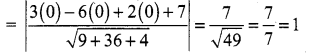Question 20.
The distance between the planes x + 2y + 3z + 7 = 0 and 2x + 4y + 6z + 7 = 0 is ……………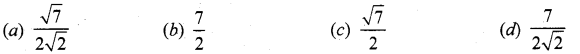Solution:
(a) $$\frac{\sqrt{7}}{2 \sqrt{2}}$$
Hint:
x + 2y + 3z + 1 = 0; 2x + 4y + 6z + 7 = 0
Multiplying 2 on both sides
2x + 4y + 6z + 14 = 0 .
a = 2, b = 4, c = 6, d1 = 14, d2 = ?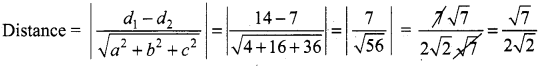Question 21.
If the direction cosines of a line are $$\frac{1}{c}, \frac{1}{c}, \frac{1}{c}$$, then ……………….
(a) c = ±3
(b) c = ± $$\sqrt{3}$$
(c) c > 0
(d) 0 < c < 1
Solution:
(b) c = ± $$\sqrt{3}$$
Hint:
We know that sum of the squares of direction cosines = 1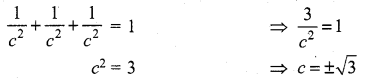Question 22.
The vector equation $$\vec{r}=(\hat{i}-2 \hat{j}-\hat{k})+t(6 \vec{j}-\hat{k})$$ represents a straight line passing through the points ……………. (a) (0, 6, -1) and (1, -2, -1) (b) (0, 6, -1) and (-1, -4, -2) (c) (1, -2, -1) and (1, 4, -2) (d) (1, -2, -1) and (0, -6, 1)
Solution:
(c) (1, -2, -1) and (1, 4, -2)
Hint:
The required vector equation is $$\vec{r}=\vec{a}+t(\vec{b}-\vec{a})$$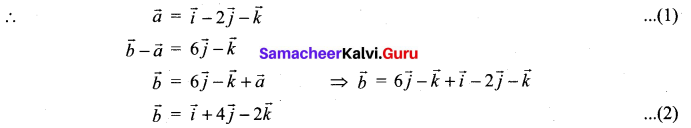From (1) and (2) The points are (1, -2, -1) and (1, 4, -2)

Question 23.
If the distance of the point (1, 1, 1) from the origin is half of its distance from the plane x + y + z + k= 0, then the values of k are ………….. (a) ±3 (b) ±6 (c) -3, 9 (d) 3, -9
Solution:
(d) 3, -9
Hint: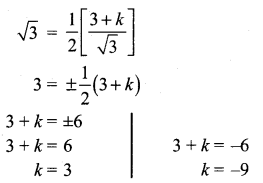Question 24.
If the planes $$\vec{r} \cdot(2 \hat{i}-\lambda \hat{j}+\hat{k})=3 \text { and } \vec{r} \cdot(4 \hat{i}+\hat{j}-\mu \hat{k})$$ = 5 are parallel, then the value of λ and µ are ……………
Solution:
(c) $$-\frac{1}{2}$$, -2
Hint: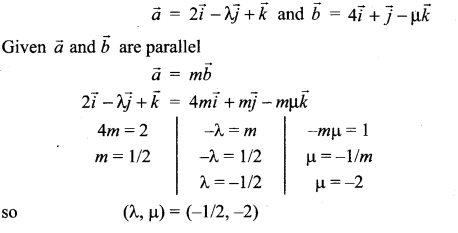Question 25.
If the length of the perpendicular from the origin to the plane 2x + 3y + λz = 1, λ > 0 is $$-\frac{1}{5}$$, then the value of λ is …………..
(a) $$2 \sqrt{3}$$
(b) $$3 \sqrt{2}$$
(c) 0
(d) 1
Solution:
(a) $$2 \sqrt{3}$$
Hint:
Given length of perpendicular from origin to the plane = $$-\frac{1}{5}$$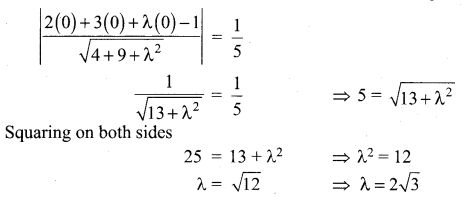### Samacheer Kalvi 12th Maths Solutions Chapter 6 Applications of Vector Algebra Ex 6.10 Additional Problems

Question 1.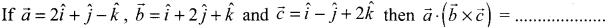(a) 6
(b) 10
(c) 12
(d) 24
Solution:
(c) 12
Hint: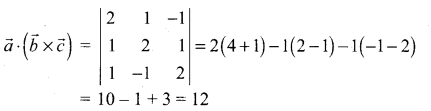Question 2.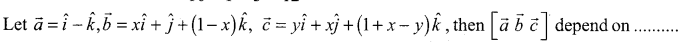(a) only x
(b) only y
(c) Neither x nor y
(d) Both x and y
Solution:
(c) Neither x nor y
Hint: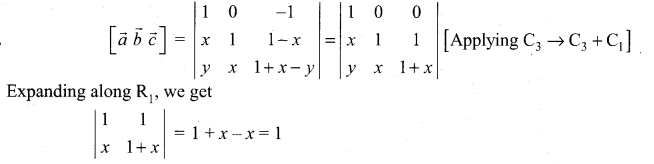Question 3.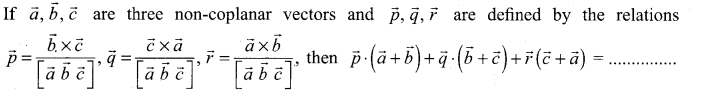(a) 0
(b) 1
(c) 2
(d) 3
Solution:
(b) 3
Hint: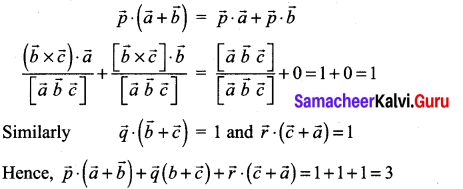Question 4.
The value of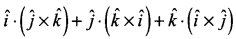= ………………
(a) 1
(b) 3
(c) -3
(d) 0
Solution:
(b) 3
Hint: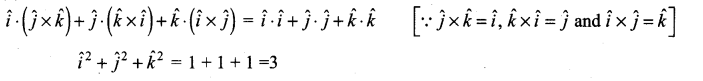Question 5.
Let a, b, c be distinct non-negative numbers. If the vectors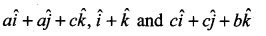lie in a plane, then c is ……………..
(a) the A.M. of a and b
(b) the G.M. of a and b
(c) the H.M. of a and b
(d) equal to zero.
Solution:
(b) the G.M. of a and b
Hint: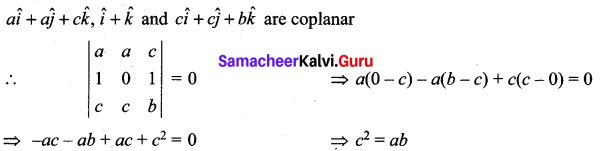Question 6.
The value of $$\hat{i} \cdot(\hat{j} \times \hat{k})+(\hat{i} \times \hat{k}) \cdot \hat{j}$$ ………….
(a) 1
(b) -1
(c) 0
(d) $$\hat{j}$$
Solution:
(c) 0
Hint: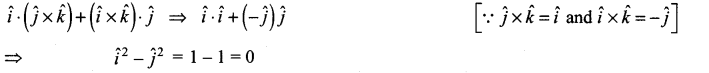Question 7.
The value of $$(\hat{i}-\hat{j}, \hat{j}-\hat{k}, \hat{k}-\hat{i})$$ is …………..
(a) 0
(b) 1
(c) 2
(d) 3
Solution:
(a) 0
Hint: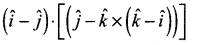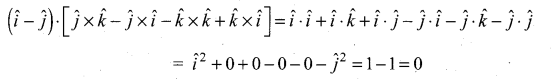Question 8.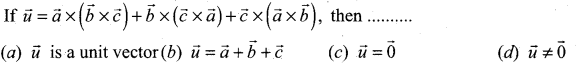Solution:
(c) $$\vec{u}=\overrightarrow{0}$$
Hint: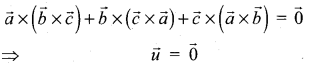Question 9.
The area of the parallelogram having a diagonal $$3 \vec{i}+\vec{j}-\vec{k}$$ and a side $$\vec{i}-3 \vec{j}+4 \vec{k}$$ is ………………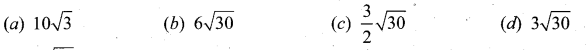Solution:
(d) $$3 \sqrt{30}$$
Solution: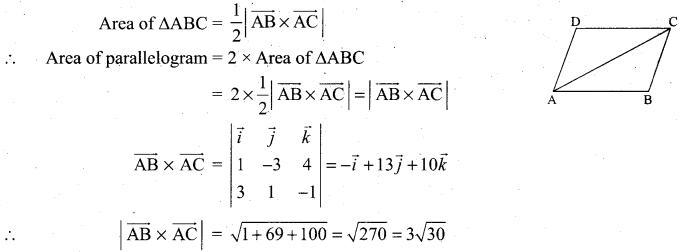Question 10.
If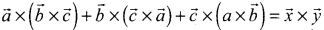, then ……………….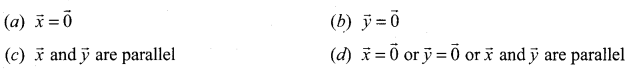Solution:
(d) $$\vec{x}=\overrightarrow{0} \text { or } \vec{y}=\overrightarrow{0} \text { or } \vec{x} \text { and } \vec{y}$$ are parallel
Hint: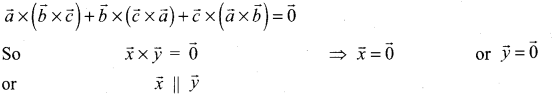Question 11.
If $$\overrightarrow{\mathrm{PR}}=2 \vec{i}+\vec{j}+\vec{k}, \overrightarrow{\mathrm{Question}}=-\vec{i}+3 \vec{j}+2 \vec{k}$$, then the area of the quadrilateral PQRS is ………………..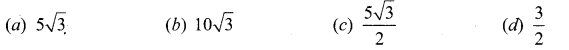Solution:
(c) $$\frac{5 \sqrt{3}}{2}$$
Hint: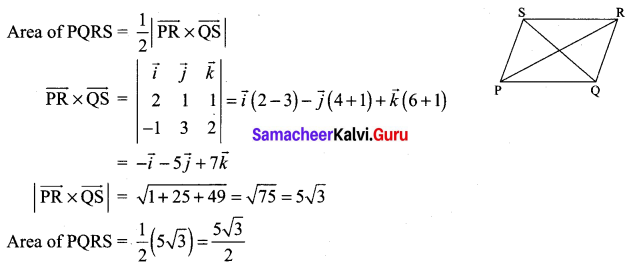Question 12.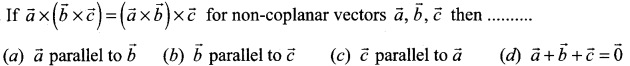Solution:
(c) $$\vec{c}$$ parallel to $$\vec{a}$$
Hint: